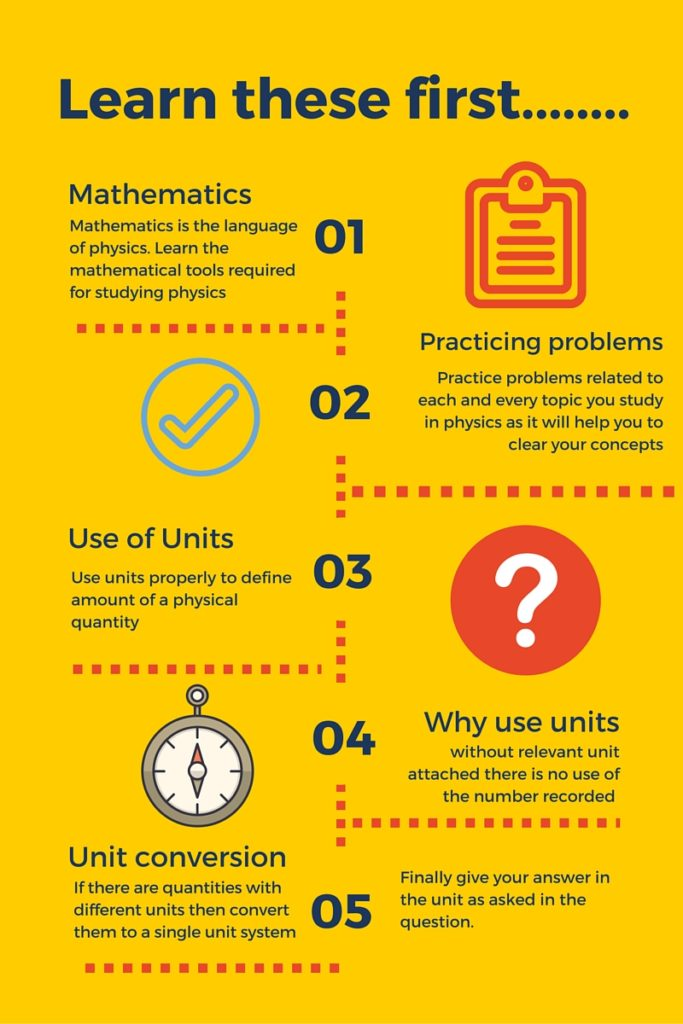# Use of units in physics

## Introduction about physics and studying the subject

When you study physics you must have appropriate skills or you would have to struggle a lot while studying the subject. In my experience students fail to learn physics not because of the science or concepts involved but mainly because of amount of mathematics involved to study the subject.

Best way to tackle this problem is to develop the skills required to study the subject. So before you start learning the subject first consider strengthening your mathematical tools required to study physics.

Now we shall discuss about the use of units in physics. We will see why we must use units while measuring physical quantities.

## Use of units in physics

This section is about the use of units in physics. So now you know physics is a quantitative subject. By this I mean to say that you cannot study physics without using numbers and units to represent physical quantities.

So let us now define physical quantities and units

Physical quantities:  All quantities in terms of which laws of physics are described, and whose measurement is necessary are called physical quantities.

Units: units are standards for measurement of physical quantities that need clear definitions to be useful.  The standard unit should be easily reproducible and internationally accepted.

So each number must be recorded with a unit otherwise it would be meaningless. To understand this further, let us consider an example where we want to record the weight of a bag of potatoes. Suppose we record a number 10 for its weight. Does this number 10 tell you how heavy is this bag of potatoes?

No, it does not tell you any such thing as there is no unit attached to this number showing weight of potato bag. You may assume weight of the bag to be as 10 gm, 10 Kg or 10 pound. In the end without unit you are not sure about the weight of the bag. To avoid confusion let us record the weight of bag as 10 kg. Now the quantity 10 Kg  of weight really tell you something and you can now imagine how heavy is this bag of potatoes.

Make it your habit to attach the unit to every physical quantity you are recording

Without relevant unit attached there is no use of the number recorded

This habit would always have a positive effect on teachers or examiners checking your answer

## Most used system of units

Most of the time we would be using SI units (International system of measurements) while studying physics. You have to use other system of units when it is mentioned in the tests or questions, otherwise SI units are convenient and widely used.

SI system of units limits the number of base units used in measurements to the following seven

SI base units
PropertyUnitSymbol
Lengthmeterm
Timesecondss
MassKilogramKg
TemperatureKelvinK
Electric currentAmpereA
Amount of substancemolemol
Luminous intensityCandelacd

In our study of mechanics we will be concerned only with the units of length, mass and time. We shall use temperature while studying thermodynamics and electric current while studying electricity and magnetism. Length, mass, time, temperature and electric current are five base units we will encounter most while studying physics. Other SI units are defined in terms of these base units.

Apart from these seven SI base quantities and units there are two supplementary quantities

PropertyUnitSymbol
##### What else now!!!

There is lot to learn about units and I am planning to do a full lesson notes on this chapter and will provide you the link in few days time.

##### What you have to do now is………..

Start learning the subject and before you start your physics course try to remember the points I have mentioned in the following graphic…If you like the article do not forget to share your views in the comments section given below. Also share the article and help us by spreading a word about us.